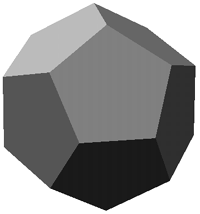# Platonic Solids

Written by Paul Bourke
December 1993

A platonic solid (also called regular polyhedra) is a convex polyhedron whose vertices and faces are all of the same type.

In two dimensions there are an infinite number of regular polygons.

In three dimensions there are just five regular polyhedra.

• Tetrahedron - made of 4 equilateral triangles
• Cube - made of 6 squares
• Octahedron - made of 8 equilateral triangles
• Dodecahedron - made of 12 regular pentagons
• Icoshedron - made of 20 equilateral triangles
In 4 dimensions there are 6 regular polytopes
• 4 simplex - made of 5 tetrahedra, 3 meeting at an edge
• Hypercube - made of 8 cubes, 3 meeting at an edge
• 16 cell - made of 16 tetrahedra, 4 meeting at an edge
• 24 cell - made of 24 octahredra, 3 meeting at an edge
• 120 cell - 120 dodecahedra, 3 meeting at an edge
• 600 cell - 600 tetrahedra, 5 meeting at an edge

### The measured properties of the 3 dimensional regular polyhedraTetrahedron Vertices: 4 Edges: 6 Faces: 4  Edges per face: 3 Edges per vertex: 3 Sin of angle at edge: 2 * sqrt(2) / 3  Surface area: sqrt(3) * edgelength^2  Volume: sqrt(2) / 12 * edgelength^3  Circumscribed radius: sqrt(6) / 4 * edgelength  Inscribed radius: sqrt(6) / 12 * edgelengthOctahedron Vertices: 6 Edges: 12 Faces: 8  Edges per face:3 Edges per vertex: 4 Sin of angle at edge: 2 * sqrt(2) / 3  Surface area: 2 * sqrt(3) * edgelength^2  Volume: sqrt(2) / 3 * edgelength^3  Circumscribed radius: sqrt(2) / 2 * edgelength  Inscribed radius: sqrt(6) / 6 * edgelengthHexahedron (cube)  Vertices: 8 Edges: 12 Faces: 6  Edges per face: 4 Edges per vertex: 3 Sin of angle at edge: 1  Surface area: 6 * edgelength^2  Volume: edgelength^3  Circumscribed radius: sqrt(3) / 2 * edgelength  Inscribed radius: 1 / 2 * edgelengthIcosahedron Vertices: 12 Edges: 30  Faces: 20  Edges per face: 3 Edges per vertex: 5 Sin of angle at edge: 2 / 3 Surface area: 5 * sqrt(3) * edgelength^2  Volume: 5 * sqrt(3 + sqrt(5)) / 12 * edgelength^3  Circumscribed radius: sqrt(10 + 2 * sqrt(5)) / 4 * edgelength  Inscribed radius: sqrt(42 + 18 * sqrt(5)) / 12 * edgelengthDodecahedron Vertices: 20  Edges: 30  Faces: 12  Edges per face: 5 Edges per vertex: 3  Sin of angle at edge: 2 * sqrt(5)  Surface area: 3 * sqrt(25 + 10 * sqrt(5)) * edgelength^2  Volume: sqrt(15 + 7 * sqrt(5)) / 4 * edgelength^3  Circumscribed radius: sqrt(sqrt(15) + sqrt(3)) / 4 * edgelength  Inscribed radius: sqrt(250 + 110 * sqrt(5)) / 20 * edgelength
"Standardised" coordinates  for the platonic solids

The solids as drawn in Kepler's Mysterium Cosmographicumand represented in stone from a neolythic settlement

Subject:
Re: Platonic Solids, intersection of three cylinders
Date:
Tue, 20 Oct 1998 08:32:24 +1000
From:
Paul Bourke <pdb@mhri.edu.au>
To:

>For my next art shows I would like to present some images of Euclidean
>three cylinders"
>For instance, the first step, in Ecole des Beaux Arts de Dijon, on november
>98, will be on a offline way.
>A CD rom for inside use will be made at this occasion.
>So, would you please tell me if you agree.